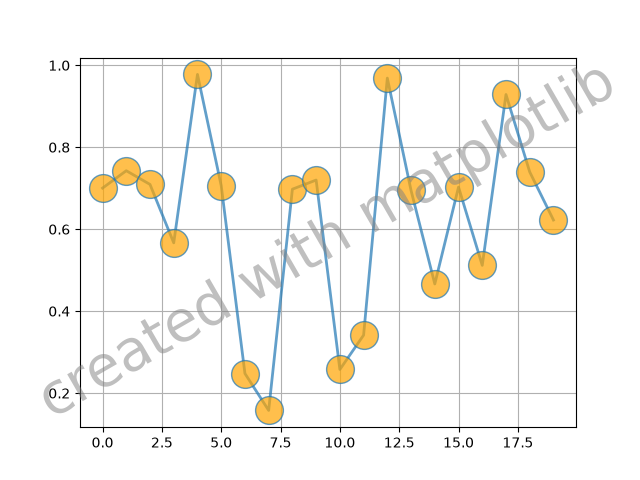# Text watermark#

A watermark effect can be achieved by drawing a semi-transparent text.

import matplotlib.pyplot as plt
import numpy as np

# Fixing random state for reproducibility
np.random.seed(19680801)

fig, ax = plt.subplots()
ax.plot(np.random.rand(20), '-o', ms=20, lw=2, alpha=0.7, mfc='orange')
ax.grid()

ax.text(0.5, 0.5, 'created with matplotlib', transform=ax.transAxes,
fontsize=40, color='gray', alpha=0.5,
ha='center', va='center', rotation=30)

plt.show()References

The use of the following functions, methods, classes and modules is shown in this example:

Gallery generated by Sphinx-Gallery